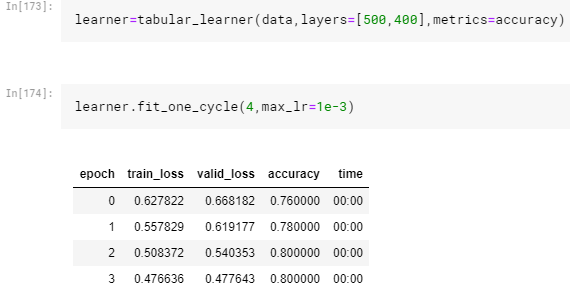# Tabular Learner gives ZeroDivisionError when fit_one_cycle is called with max_lr slice

Hi,
I am trying to work with the Titanic Dataset from kaggle using the tabular learner. It doesn’t show any error if fit_one_cycle is called with a fixed max_lr.I tried fine tuning my model with techniques from lesson 1, by finding learning rate.
But it shows ZeroDivisionError if max_lr is sliced between a range.

I’m obviously doing something wrong here.
Can anyone point them out?

Thanks a bunch

Hey Rafid,

Welcome to the forums! The tabular models are all one group so we cannot do the differential learning rates. They also do not apply transfer learning either. That’s why you get that error. Does this make sense?

2 Likes

Thank you, Zachary.

I don’t understand the concept of grouping models. Could you point out where I can read up on it?

Also how do I find the optimal learning rate for my tabular model? Can I use learner.recorder.plot() in this case?

Sure! So essentially what I mean by this are the layer groups. When we have a ResNet model, the initial batch of layers where the tensor images are quite small (The first 1/3 or so) are considered one group, then the next chunk of layers up til the end is another, and finally the last few layers are the last group. These three groups allow for the use of `fit_one_cycle` , as we can train each group at different rates. This allows for a faster learning rate in places where there will not be much difference between the layers, until we get to the larger images in the model and we can train for slower there. Let me know if there still questions on that, I can try to find a visual, I believe Jeremy has one in the lectures, I can find that for you.

On the learning rate for the tabular model, absolutely! You call fit() and pass in a learning rate, as in this case, `fit` and `fit_one_cycle` will both do the exact same thing here, due to those layer groups stated above. If you want to see what could be the best learning rate narrowed down, when you call `learn.recorder.plot()`, you can pass in `suggestion`, which will show a red dot where a potential good LR is. For example:

`learn.recorder.plot(suggestion=True)`

It is always a good habit to pass in the learning rate when you can, just whether you pass in the tuple of learning rates (the pair that you tried) or an individual one depends on the model!

Does this all make sense?

3 Likes

Are you referring to Jeremy’s illustration explaining why learning rate is gradually increased at first and decreased again after reaching max_lr?

I think I understand now. I’ll try your suggestions out.
Thank you so muchYep! That’s is correct.

So I think I have understood the different approaches to use the `max_lr` param in `fit_one_cycle` because sometimes throughout the course lectures it’s used with just one value, sometimes with the `slice(..)` function.
Using the `slice(..)` function then only makes sense for learner-models with different layer-groups (mainly convolutional models) because models like Tabularlearner only use on learning rate?

Close, mostly due to the fact of how we aren’t using pre-trained models. If we had a pretrained tabular model we’d want to split our model into two groups and do like we do for images, but since not we train everything at the same learning rate!1 Like

Hi [muellerzr],

Thank you for your replies to [abyaadrafid] I was having the same issue and they helped out a lot!

You mentioned that tabular models do not apply transfer learning either, but could it be possible to do this? Idea being that I could train a model on a dataset generated by an analytical model and then use transfer learning to re-train it further on an experimental dataset.

This would be useful in situations where the equations that we use to make mathematical models do not capture completely the trends in experimental results, yet the nature of their inputs would be identical.

In my head at least it feels like a useful application of transfer learning (if possible) as experimental data of course is much harder/expensive to source than analytical data.

Any comment would be appreciated, thanks!

Sam

Hi Samuel! It’s possible by using the embeddings (experimental) here is a thread with a few techniques: Tabular Transfer Learning and/or retraining with fastai

For a place to start@samph4

1 Like

Thankyou @muellerzr!

I’ll have a look